# Cross section

The cross-section ABCD of a swimming pool is a trapezium. Its width AB=14 meters, depth at the shallow end is 1.5 meters, and at the deep end is 8 meters. Find the area of the cross-section.

Correct result:

A =  66.5 m2

#### Solution: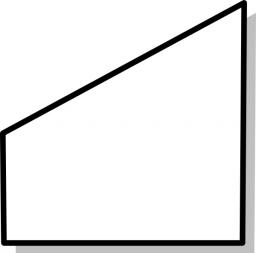We would be pleased if you find an error in the word problem, spelling mistakes, or inaccuracies and send it to us. Thank you!Math student
????that's  correctGeorge
a fiberglass pool that is 12.3 wide and 23.9 long and is 3'9-5'11 deep, would need how many panels?

2 years ago  1 LikeMath student
Thank youMath student
Not clearMath student
it is not my problem diagram is right and sum is wrongMath student
Correct. Thank youTips to related online calculators
Looking for help with calculating arithmetic mean?
Looking for a statistical calculator?

#### You need to know the following knowledge to solve this word math problem:

We encourage you to watch this tutorial video on this math problem:

## Next similar math problems:

• Here isHere is a data set (n=117) that has been sorted. 10.4 12.2 14.3 15.3 17.1 17.8 18 18.6 19.1 19.9 19.9 20.3 20.6 20.7 20.7 21.2 21.3 22 22.1 22.3 22.8 23 23 23.1 23.5 24.1 24.1 24.4 24.5 24.8 24.9 25.4 25.4 25.5 25.7 25.9 26 26.1 26.2 26.7 26.8 27.5 27.6 2
• Swimming pool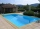A swimming pool 30 meters long is filled with water to a depth of 1 meter at the shallow end and 5 meters at the deep end and abcd the vertical area of the pool has the shape of a trapezium with the area given by S(abcd)= 1/2 (ab + cd) x ad. What is the a
• PoolIf water flows into the pool by two inlets, fill the whole for 19 hours. The first inlet filled pool 5 hour longer than the second. How long pool take to fill with two inlets separately?
• Brick wall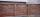Garden 70 m long and 48 m wide should surround with wall 2.1 meters high and 30 cm thick. Wall will be built on the garden ground. How many will we need bricks if to 1 m³ is required approximately 300 bricks?
• Top of the towerThe top of the tower has the shape of a regular hexagonal pyramid. The base edge has a length of 1.2 m, the pyramid height is 1.6 m. How many square meters of sheet metal is needed to cover the top of the tower if 15% extra sheet metal is needed for joint
• PitPit has shape of a truncated pyramid with rectangular bases and is 0.8 m deep. The length and width of the pit is the top 3 × 1.5 m bottom 1 m × 0.5 m. To paint one square meter of pit we use 0.6 l of green colour. How many liters of paint is needed when
• Chocolate rollThe cube of 5 cm chocolate roll weighs 30 g. How many calories will contain the same chocolate roller of a prism shape with a length of 0.5 m whose cross section is an isosceles trapezoid with bases 25 and 13 cm and legs 10 cm. You know that 100 g of this
• Dusana) Dusan break two same window, which has triangular shape with a length of 0.8 m and corresponding height 9.5 dm. Find how many dm2 of glass he needs to buy for glazing of these windows. b) Since the money to fix Dusan has not, must go to the paint job
• Sunflower Field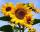The trapezoidal sunflower field is located between two parallel paths which are spaced 230 meters apart. The lengths of the parallel sides of the field are 255 m and 274 m. How many tons of sunflower will come from this field if the hectare yield is 2.25
• OrchardRoute passes trapezoidal orchard perpendicular to the parallel sides. It is 80 cm wide. The lengths of the bases are in the ratio 5:3 and the length of the longer base to the length of the path is in the ratio 5:6. How many square meters occupies the rout
• Railways - golden parachutes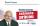As often happens in Slovakia habit, the state's financial institution which takes from poverty and gorilas give. A hardworking punishing taxes. Let's look at a short work of director Railway Company ZSSK - Mgr . P. K. : 18 months 'work' as director reduct
• PoolThe swimming pool is 10 m wide and 8 m long and 153 cm deep. How many hectoliters of water is in it, if the water is 30 cm below its upper edge?
• Roof tilesThe roof has a trapezoidal shape with bases of 15 m and 10 m, height of roof is 4 meters. How many tiles will need if on 1 m2 should be used 8 tiles?
• Speed of Slovakian trainsRudolf decided to take the train from the station 'Ostratice' to 'Horné Ozorovce'. In the train timetables found train Os 5409 : km 0 Chynorany 15:17 5 Ostratice 15:23 15:23 8 Rybany 15:27 15:27 10 Dolné Naštice 15:31 15:31 14 Bánovce nad Bebravou 15:35 1Road embankment has a cross section shape of an isosceles trapezoid with bases 5 m and 7 m, and 2 m long leg. How many cubic meters of soil is in embankment length of 1474 meters?Calculate the surface of the quadrilateral prism ABCDA'B'C'D 'with the trapezoidal base ABCD. The height of the prism is 12 cm; ABCD trapezoidal data: AB base length is 8 cm, CD base length is 3 cm, BC arm length is 4 cm, and AC diagonal length is 7 cm. LQuestion No. 1: The prism has the dimensions a = 2.5 cm, b = 100 mm, c = 12 cm. What is its volume? a) 3000 cm2 b) 300 cm2 c) 3000 cm3 d) 300 cm3 Question No.2: The base of the prism is a rhombus with a side length of 30 cm and a height of 27 cm. The heig